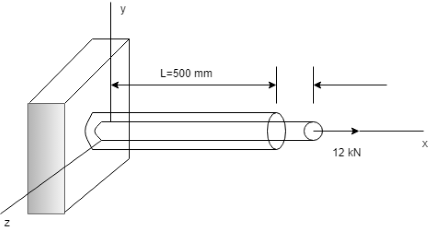# A 16 mm diameter cylindrical bar is subjected to a load of 12 KN. Under this load the beam...

## Question:

A 16 mm diameter cylindrical bar is subjected to a load of 12 KN. Under this load the beam extended 300{eq}\mu {/eq}m and the diameter reduces by 2.4{eq}\mu {/eq}m. Determine the young's modulus and Poisson's ratio for the material?## Lateral and Longitudinal Strain:

The strain takes place in the perpendicular direction of the applied load on the member is called lateral strain whereas the strain takes place in the direction of the applied load on the member is called longitudinal strain.

Given Data:

• The diameter of the cylinder bar is: {eq}d = 16\;{\rm{mm}} {/eq}
• The axial load subjected to the bar is: {eq}P = 12\;{\rm{kN}}{/eq}
• Th...

Become a Study.com member to unlock this answer! Create your account